# GRE Subject Test: Chemistry : Titrations

## Example Questions

← Previous 1

### Example Question #1 : Titration Curves

Which of the following pH values is an acceptable equivalence point for a weak base being titrated by a strong acid?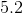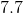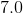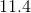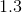Explanation:

The equivalence point is the point during a titration when there are equal equivalents of acid and base in the solution. Since a strong acid will have more effect on the pH than the same amount of a weak base, we predict that the solution's pH will be acidic at the equivalence point. 5.2 and 1.3 are both acidic, but 1.3 is remarkably acidic considering that there is an equal amount of base in the solution. As a result, 5.2 is a more appropriate answer.

The equivalence point for a strong acid and strong base will be 7.0. When one of the compounds is weak, however, it will dissociate less than its strong counterpart. In our case, the base will dissociate less than the acid. The acid, thus, contributes more to the pH character of the solution.

### Example Question #1 : Titration Curves

A weak acid is slowly titrated with a strong base. Where on the titration curve would the solution be the most well-buffered?

The equivalence point

When the amount of base added equals the amount of acid in the solution.

The half equivalence point

When no base has been added

The half equivalence point

Explanation:

At the half equivalence point, the concentration of acid in the solution is equal to the concentration of the conjugate base in solution. At this point, the graph shows a line that is near horizontal. This means that base or acid could be added and the pH of the solution would change very slowly.

Remember that pH is on the y-axis of the titration curve; thus a near-horizontal line will signify a region where pH is most stable. At the half equivalence point, pH = pKa.

### Example Question #2 : Titration Curves

Which of the following curves represents the titration of a strong base by a strong acid?

Explanation:

Since we are adding acid to a base, the pH must decrease. The initial base will have a high pH, while the final acid will have a low pH; the right of the curve must be lower than the left. In addition, the pH does not change very rapidly until the equivalence point is reached, hence the curve must show little initial change followed by a rapid change.

### Example Question #1 : Titrations

Which of the following is an indication of when a reaction has reached the equivalence point.

When the volume of both reactants are equal

The end point of a titration

When there is a color change

Moles of titrant equals moles of analyte

Moles of titrant equals moles of analyte

Explanation:

The equivalence point is the point in a titration at which the number of moles of titrant equals the number of moles of analyte. The endpoint in a titration is when the color of the reaction changes to that of the desired (often neutral) pH. However this is not the same as the equivalence point, which is a stoichiometric value. Indicators may change colors several times throughout a neutralization reaction, thus this is not an accurate attribute of the reaction to use as the equivalence point. Furthermore, the concentrations of titrant and the analyte are rarely equivalent, and there could be differing numbers of ionizable groups on each species.

### Example Question #2 : Titrations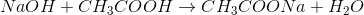Considering the given chemical reaction, determine the number of moles of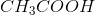in a 20mL solution if it takes 19.00mL of a 0.0500M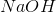solution to reach the endpoint of a titration.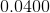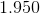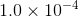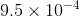Explanation:andreact in a 1:1 mole ratio. Therefore the number of moles ofat the end point of the reaction equals to the number of moles of. We can use the concentration as a conversion factor to determine the number of moles reacted.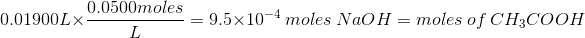### Example Question #1 : TitrationsConsidering the given chemical reaction, determine the concentration ofin a 40.00mL solution if it takes 25.00mL of a 0.0350Msolution to reach the endpoint of a titration.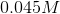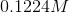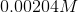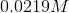Explanation:andreact in a 1:1 mole ratio. Therefore the number of moles ofat the end point of the reaction equals to the number of moles of.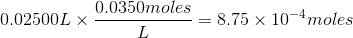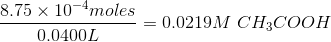### Example Question #4 : Titrations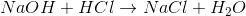Considering the given neutralization reaction, what is the concentration of the sodium chloride solution if it takes 20.00mL of 0.045M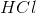to neutralize 20mL of thesolution?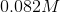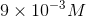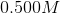Explanation:

Based on the equation given, we know the thatreacts within a 1:1 mole ratio. We can use the molar concentration given as a conversion factor to determine the number of moles ofused which will be equal to the number of moles ofused.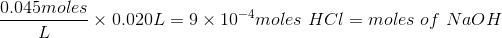To determine the molar concentration ofused, we can simply use the following equation: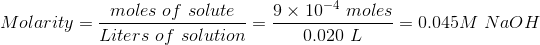We have determined that the concentration ofused is equal to the concentration ofused.

### Example Question #2 : TitrationsA vinegar sample was determined by a titration withto have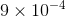moles  ofin a 2.00 grams of vinegar. What would be the percent mass of this vinegar solution?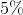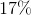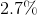Explanation:
We must first calculate the molecular weight of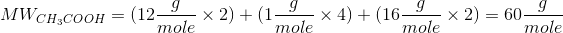We can calculate the grams ofusing the molecular weight calculated as a conversion factor: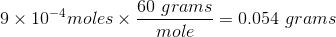Finally, we can calculate the percent mass using the total grams of vinegar solution: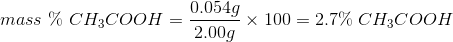### Example Question #1 : Titrations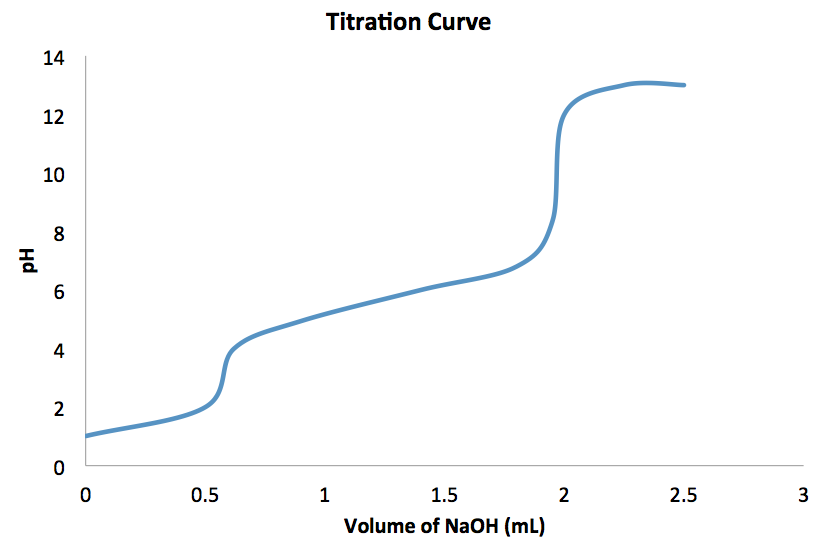Based on the pH titration curve provided, how many acidic protons are in this acid?

Three

Four

It cannot be determined from the graph

Two

One

Two

Explanation:

The equivalence point is the point in a titration at which the number of moles of titrant species equals the number of moles of analyte. For a polyprotic acid there are multiple equivalence points because there are more than one acidic proton in one molecule of the acid. A titration curve has as many equivalence points as the number of protons that may be neutralized by the interaction with a base. In this titration curve there are two equivalence points. The equivalence points occur when the graph has a very steep slope, and involve very large changes in pH with additions of small amounts of base. For this graph, the equivalence points occur at about pH 3.5 and 10.

### Example Question #1 : Titrations

What volume in liters of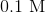is needed in the titration of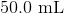of a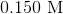(acetic acid) solution to reach the equivalence point?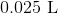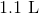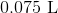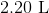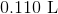Explanation:

The equivalence point in a titration is the point in which the number of moles of titrant equals to the number of moles of analyte: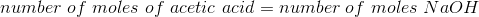We need to determine the number of moles of acetic acid we are dealing with: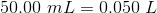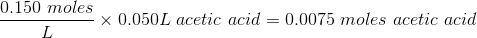Therefore, at the equivalence point there are also 0.0075 moles ofin the solution. In order to determine the number of moles ofin solution, we must use the concentration ofas a conversion factor to determine the volume added to the acetic acid solution.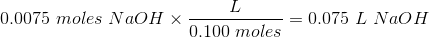← Previous 1

### All GRE Subject Test: Chemistry Resources